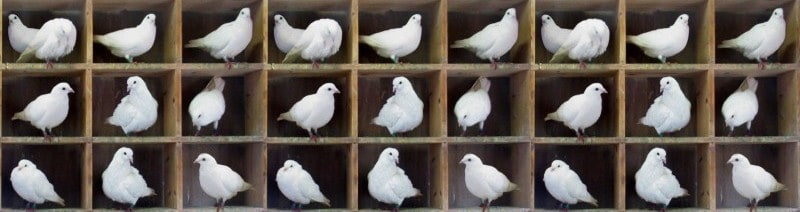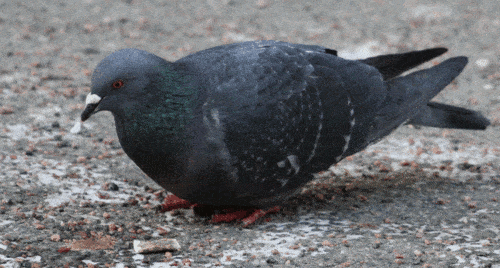# Pigeonhole principleSome time ago we’ve received a pretty interesting question from one of our visitors. It sounds as follows:

Let $A$ be any set of $20$ distinct integers chosen from the arithmetic progression $1, 4, 7, …, 100$.
Prove that there must be two distinct integers in $A$ whose sum is $104$.

We’ve answered with a video explanation. Check it on our channel:This problem can be solved with the help of so called Pigeonhole principle. It can be stated simply as follows: if $m$ pigeons are put into $m$ pigeonholes, then there is an empty hole if and only if there’s a hole with more than one pigeon.Maybe, it sounds trivial this way, yet some very interesting results can be shown through this approach. For example, applying pigeonhole principle, we can show that at any given time in New York there live at least two people with the same number of hairs. You can find the proof on the Internet, if you like. Later we’ll provide more formal statement. Let’s return to our task.

The numbers in the sequence are:

\begin{aligned} 1, 4, 7, 10, 13, 16, 19, 22, 25, 28, 31, 34, 37, 40, 43, 46, 49, 52, 55, 58, 61, 64, 67, 70, 73, 76, 79, 82, 85,&\\ 88, 91, 94, 97, 100\end{aligned}

Note that the numbers $1, 4, 7, …, 100$ can be represented in the form $3n + 1$ for $n = 0, 1, …, 33$ (for $n=0$ we have $1$, for $n=1$ we get $4$ and so on).

We want to find pair of numbers $3n+1$, $3m+1$ ($m,n=0, 1, .., 33$) such that their sum equals:

$$3n+1+3m+1=104$$

Simplifying this, we obtain the following:

$$3m+3n=102$$

$$n+m = 34$$

Evidently $n = 0$ (which corresponds to number $1$) and $n = 17$ (number $52$) do not help. This is because the largest number in sequence is $100$ and so we get:

$$1+100=101<104$$

As for $52$, this number can yield desired $104$ only in pair with $52$ , but, according to the statement, we need distinct numbers.

The other $32$ numbers form $16$ pairs with the required sum. These pairs are:

$4$ and $100$
$7$ and $97$
$10$ and $94$
$13$ and $91$
$16$ and $88$
$19$ and $85$
$22$ and $82$
$25$ and $79$
$28$ and $76$
$31$ and $73$
$34$ and $70$
$37$ and $67$
$40$ and $64$
$43$ and $61$
$46$ and $58$
$49$ and $55$

Now we use Pigeonhole principle. As we know, it states that if $n$ items are put into $m$ pigeonholes with $n > m$, then at least one pigeonhole must contain more than one item. We have $16$ pairs fitting the sum requirement. So if we take at least $19$ numbers (and due to the statement we take $20$ numbers) then we surely to get two from the same pair (because if in the worst case we chose $52, 1$ (which don’t form pairs, as we said above) and $16$ numbers from each pair then there are already $18$ numbers .

None of these numbers add up to $104$ as only one number has been selected from the $16$ “good” pairs and $1$ and $52$ do not combine with any other number to give the required sum of $104$. However, there’re still  2 more numbers left to make up $20$ elements for the set $A$. These necessarily have to be two second numbers from two pairs. Thus, the set of $20$ integers $A$ has two pairs of numbers whose sum is $104$
and there will surely be two distinct integers whose sum is $104$.

Hopefully, now you have general idea about how pigeonhole principle works and, actually, why it’s called so. Here’s more formal way to state it:

Let $|A|$ denote the number of elements in a finite set $A$. For two finite sets $A$ and $B$, there exists a one-to-one correspondence if and only if $|A| = |B|$.

Imagine number of elements in $A$ as pigeons, and number of elements in $B$ as holes. Pigeons are alone each in its hole if and only if there’re as many holes as pigeons. So there again.